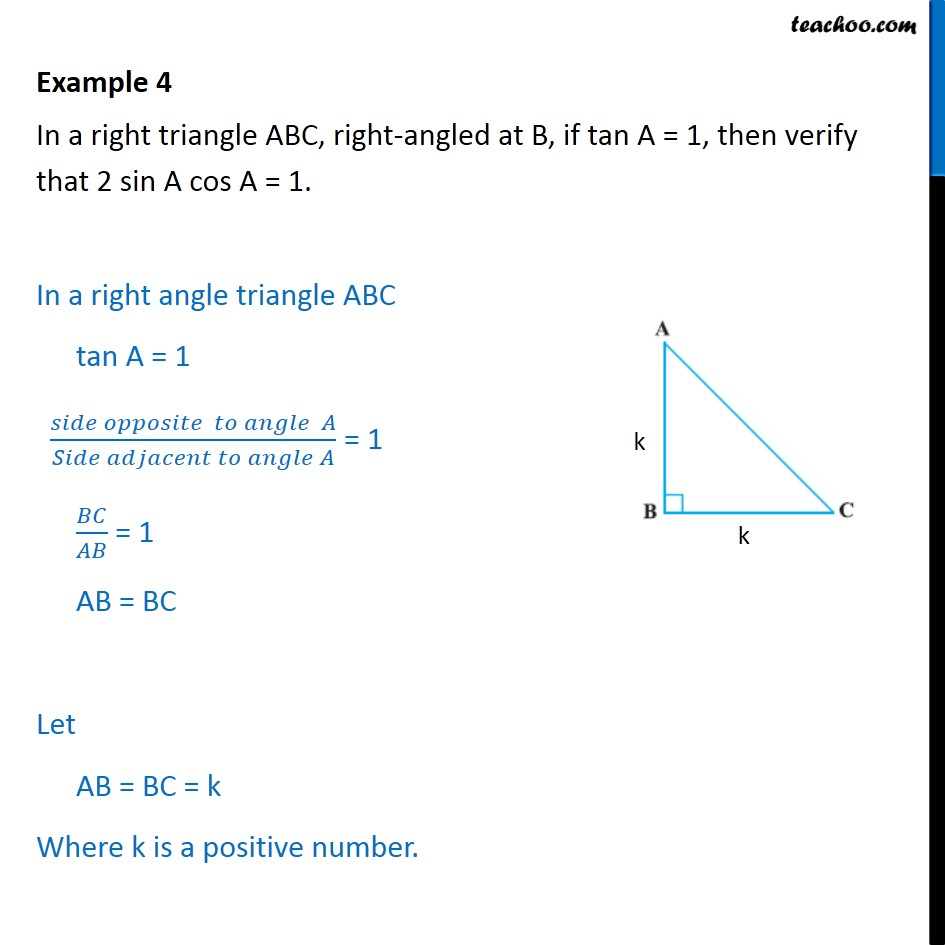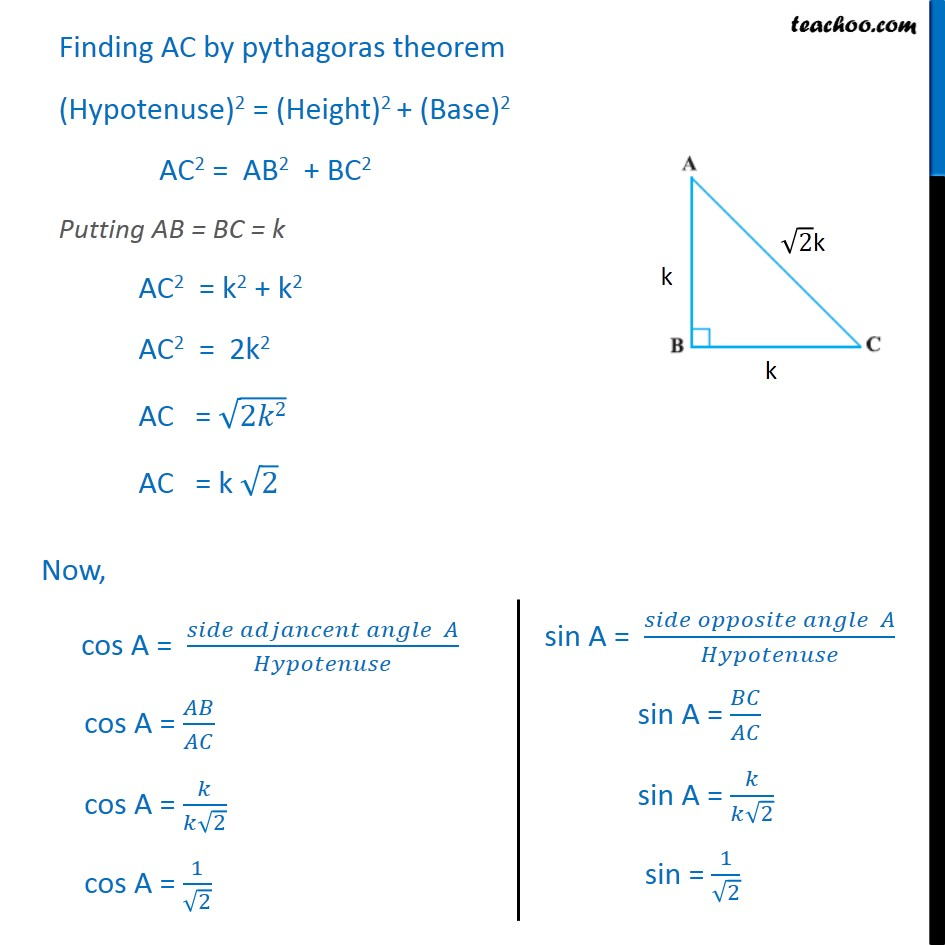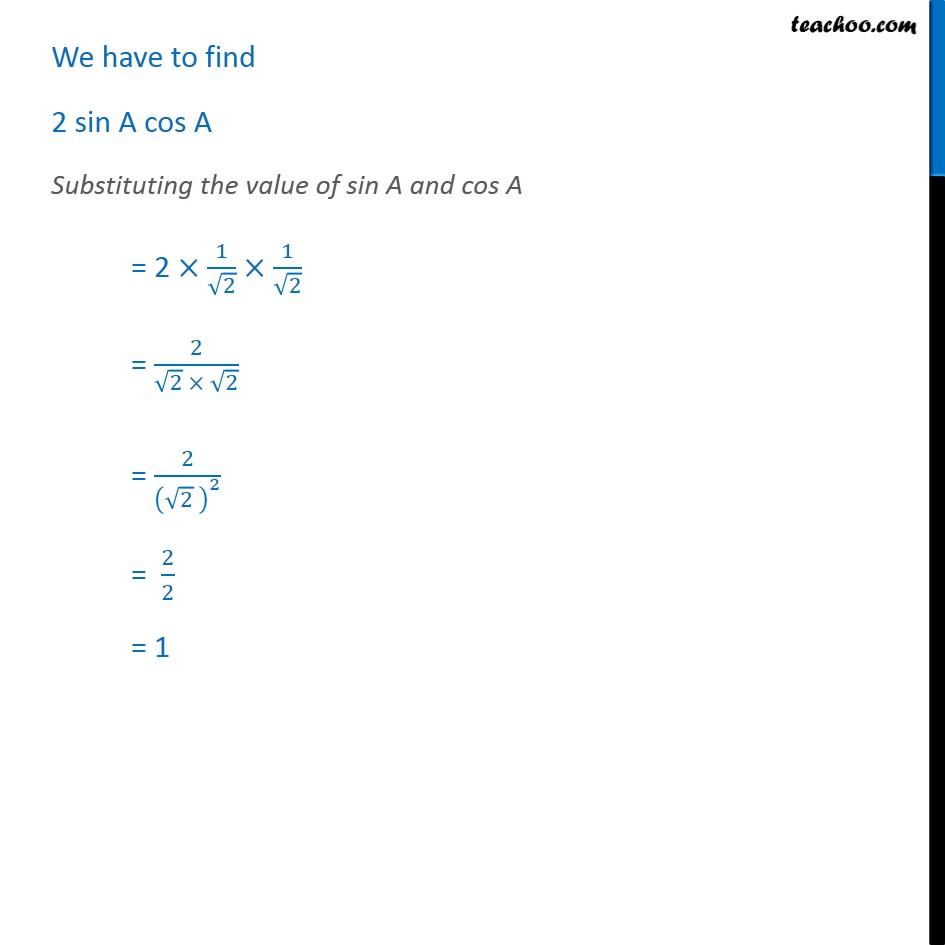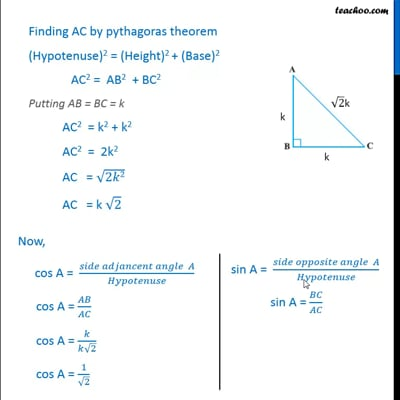Examples

Chapter 8 Class 10 Introduction to Trignometry
Serial order wiseThis video is only available for Teachoo black users

Solve all your doubts with Teachoo Black (new monthly pack available now!)

### Transcript

Example 4 In a right triangle ABC, right-angled at B, if tan A = 1, then verify that 2 sin A cos A = 1. Finding AC by pythagoras theorem (Hypotenuse)2 = (Height)2 + (Base)2 AC2 = AB2 + BC2 Putting AB = BC = k AC2 = k2 + k2 AC2 = 2k2 AC = √2𝑘2 AC = k √2 We have to find 2 sin A cos A Substituting the value of sin A and cos A = 2 ×1/√2×1/√2 = 2/(√2 × √2) = 2/(√2 )^2 = 2/2 = 1